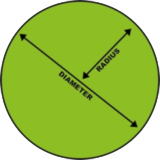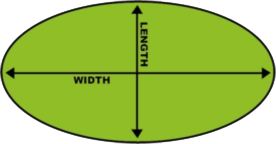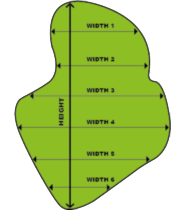# How to measure your new lawn area

## This guide helps you work out the metres of instant lawn you need

Once you have the correct m2 contact us and we will give you a quote. It is advisable to allow extra turf when ordering as you will have some wastage particularly if your lawn is of an irregular shape.

## Comes With A 10-Year Product Warranty

### Measuring a Rectangle

• Measure the height and width of the square or rectangular area in metres
• To calculate the amount of turf required, multiply height x width
• Example:
• - Height 6 m; Width 15 m
• - 6 m x 15 m= 90 m2### Measuring a Circle

• Measure the diameter of the circular area in metres
• To calculate the amount of turf required, use this simple formula. r = radius d = diameter
• r x r x 3.14
• Example:
• - Diameter 13 m radius 6.5 m
• - 6.5 m x 6.5 m x 3.14 = 132.7 m### Measuring an Oval

• Measure the length and width of the oval area in metres
• To calculate the amount of turf required, multiply length x 0.80 then by width
• Example:
• - Length 19 m; Width 10 m
• - 19 m x 0.80 = 15.2 m
• - 15.2 m x 10 m = 152 m2### Measuring unusual shapes

• Measure the length and width of the oval area in metres.
• To calculate the amount of turf required, multiply length x 0.80 then by width.
• Example:
• - Length 19 m; Width 10 m
• - 19 m x 0.80 = 15.2 m
• - 15.2 m x 10 m = 152 m2